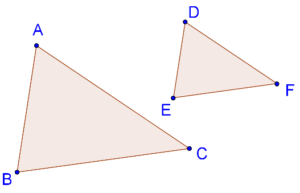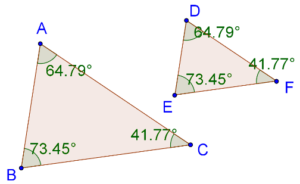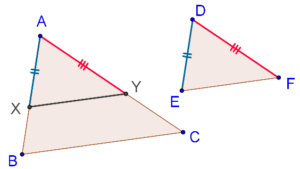# SSS Criterion in Triangles

Go back to  'Similar-Triangles'

We saw that if two triangles are equi-angular, then they are similar. Can we say that if the sides of two triangles are (respectively) proportional, they will be similar? Yes, we can.

SSS Criterion: If the three sides of one triangle are respectively proportional to the three sides of another, then the two triangles are similar.

This essentially means that any such pair of triangles will be equi-angular. Consider the following figure, in which the sides of two triangles ($$\Delta ABC$$ and $$\Delta DEF$$) are respectively proportional:That is, it is given that:

$\frac{{AB}}{{DE}} = \frac{{BC}}{{EF}} = \frac{{AC}}{{DF}}$

Can we say that these two triangles will be equi-angular? The SSS criterion says that we can. Indeed, if we measure the angles in each triangle, we will find this to be true, as the following figure shows:Let us now discuss the proof of the SSS criterion.

Proof: Suppose that AB > DE. Then AC will also be greater than DF (why). Now:

1.  Take a point X on AB such that AX = DE.

2.  Take a point Y on AC such that AY = DF.

Join XY:It is given that

$\frac{{DE}}{{AB}} = \frac{{DF}}{{AC}}$

Thus,

$\frac{{AX}}{{AB}} = \frac{{AY}}{{AC}}$

Using the converse of the BPT, this implies that XY || BC. Thus, $$\Delta AXY$$ ~ $$\Delta ABC$$. This further means that:

$\frac{{AX}}{{AB}} = \frac{{AY}}{{AC}} = \frac{{XY}}{{BC}}$

But we also have:

$\frac{{EF}}{{BC}} = \frac{{AY}}{{AC}} = \frac{{AX}}{{AB}}$

Thus,

\begin{align}& \frac{{XY}}{{BC}} = \frac{{EF}}{{BC}}\\ &\Rightarrow XY = EY \end{align}

Now, by the SSS criterion, $$\Delta DEF$$ ≡ $$\Delta AXY$$, while we already have $$\Delta AXY$$ ~ $$\Delta ABC$$. Thus, $$\Delta DEF$$ is similar to $$\Delta ABC$$. This concludes our proof.

Learn math from the experts and clarify doubts instantly

• Instant doubt clearing (live one on one)
• Learn from India’s best math teachers
• Completely personalized curriculum